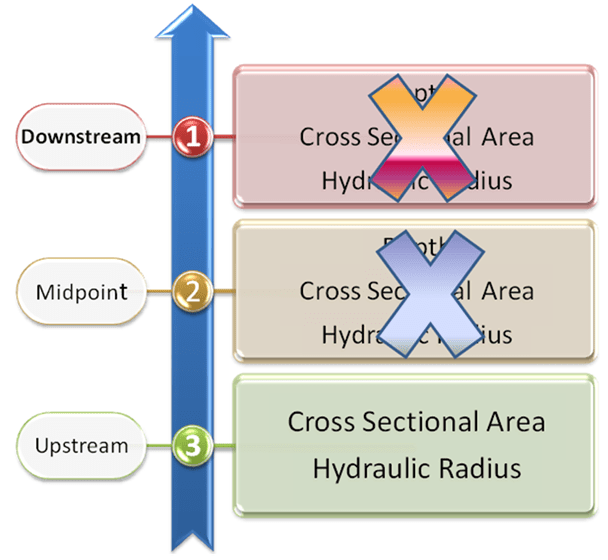#INFOSWMM

# Link Simulated Parameters used in either the Normal Flow or St Venant Equation of SWMM 5

Subject:  Link Simulated Parameters used in either the Normal Flow or St Venant Equation of SWMM 5

StVenant equation – this is the link attribute data used when the StVenant Equation is used in SWMM 5.  Simulated Parameters from the upstream, midpoint and downstream sections of the link are used.

Normal Flow Equation – this is the link attribute data used when the Normal Flow Equation is used in SWMM 5. Only simulated parameters from the upstream end of the link areused if the normal flow equation is used for the time step.